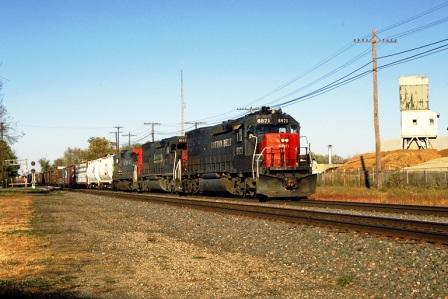# KB241: 4-digit Addressing Formula

This article was last updated on Jan. 13, 2012, 10:46 a.m. | Print Article | Leave FeedbackZephyr and systems using the DT400 will automatically program 4-digit address' with no math required.

Those using other systems must manually program a 4-digit address.

If you want to program a 4 digit address you simply cannot take the desired locomotive address and split the number and stick it into CV17 & CV18.

The following example will NOT work: Locomotive 2345 CV17 = 23, CV18 = 45 – This isn’t how 4 digit addressing works.

This method WILL work:

Here is a simple method for figuring out what values belong in CV17 & CV18:

The desired locomotive 4 digit address is DIVIDED by 256.

Add 192 to the whole number. The sum is entered into CV17.

MULTIPLY the whole number by 256 (the number before adding 192).

The result is them SUBTRACTED from the desired locomotive address.

EXAMPLES
: 2345 -- Desired Locomotive address 2345 / 256 = 9.16015625 9 + 192 = 201 CV17 will be 201 9 * 256 = 2304 2345 – 2304 = 41 CV18 will be 41 7821 -- Desired Locomotive address 7821 / 256 = 30.55078125 30 + 192 = 222 CV17 will be 222 30 * 256 = 7680 7821 – 7680 = 141 CV18 will be 141 Remember to set CV29 to the correct value!

When you are setting CV17 & 18 individually to program a 4-digit address, you need to "set" CV29 to 'activate' the newly programmed address.

CV29 comes factory programmed to 06.

The value of 06 equals 2 digit address [which is the value in CV1 (AD1)], Analog ON, NDOT [Normal Direction of Travel] Forward.

To use the values in CV17 & 18, CV29 needs to be changed.

Common values are: 038 4 digit address, Analog on, NDOT Forward 039 4 digit address, Analog on, NDOT Reverse 034 4 digit address, Analog off, NDOT Forward 035 4 digit address, Analog off, NDOT Reverse In these examples, the current value in CV29 was taken and 32 added to it.

Since you have created a 4-digit address, 038 or 039 or 034 or 035 are chosen to activate the 4-digit address.
Choosing NDOT in this example usually means a value of 038 or 034, but if you like railroads that ran their locomotives long nose forward (such as the Southern Railway), then a value of 039 or 035 is used.

"Analog" refers to setting the decoder to be able to operate on DC powered model railroads; many users choose to turn Analog "off".

There also are many 4 digit calculators on the web that will do the math for you.